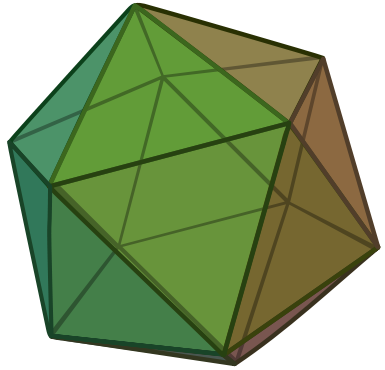# Icosahedron of ResistorsOne of my first physics problems.

Each edge of an icosahedron is a 1­ $\Omega$ resistor. If the effective resistance between two adjacent vertices can be represented as $\frac {a}{b} \Omega$ where $a$ and $b$ are co-prime positive integers, find $a + b$.

An icosahedron consists of 20 equilateral triangles. It has 12 vertices and 30 edges, with 5 edges meeting at each vertex.

Image credit: Wikipedia DTR

×

Problem Loading...

Note Loading...

Set Loading...